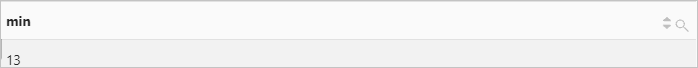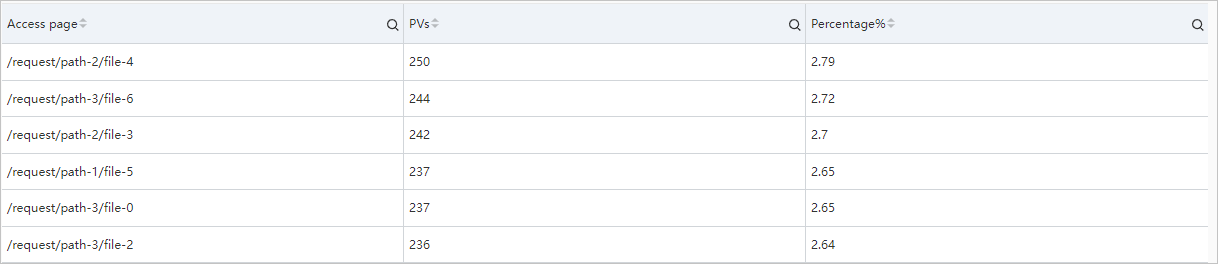A subquery is a query in which a SELECT statement is nested inside another SELECT statement. You can use subqueries to meet complex analysis requirements.

## Syntax

Specify the FROM clause in a SELECT statement.

``* | SELECT key FROM (sub_query)``
Notice
• You must enclose the subquery statement in the FROM clause in parentheses `()`.
• If you want to analyze log data in the current Logstore, you must specify the keyword `FROM log`.

## Examples

### Example 1

Calculate the number of page views (PVs) by request method and obtain the minimum number of PVs.

• Query statement
``````* |
SELECT
min(PV)
FROM  (
SELECT
count(1) as PV
FROM      log
GROUP BY
request_method
)``````
• Query and analysis results### Example 2

Calculate the ratio of the PVs in the current hour to the PVs in the same time period on the previous day. The time range for the query is 1 hour (on the hour). 86400 indicates the result of the current time minus 86400 seconds, which is equivalent to 1 day. log indicates the name of the Logstore.

• Query statement
``````* |
SELECT
diff  AS today,
diff  AS yesterday,
diff  AS ratio
FROM  (
SELECT
compare(PV, 86400) AS diff
FROM      (
SELECT
count(*) AS PV
FROM          log
)
)``````
• Query and analysis results• 3337.0 indicates the PVs in the current hour. Example: the PVs from 14:00:00 to 15:00:00 on December 25, 2020.
• 3522.0 indicates the PVs in the same time period on the previous day. Example: the PVs from 14:00:00 to 15:00:00 on December 24, 2020.
• 0.947473026689381 indicates the ratio of the PVs in the current hour to the PVs in the same time period on the previous day.

### Example 3

Calculate the number of PVs on each page and the percentage of the PVs on each page to the total PVs.

• Query statement
``````* |
SELECT
request_uri AS "Access page",
c as "PVs",
round(c * 100.0 /(sum(c) over()), 2) AS "Percentage%"
FROM  (
SELECT
request_uri AS request_uri,
count(*) AS c
FROM      log
GROUP BY
request_uri
ORDER BY
c DESC
)``````
• Query and analysis results